# Probability + reason - math problems

#### Number of problems found: 61

• Error rate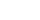The exam has 6 questions. Students have an error rate of 20% and can have a maximum of 1 question wrong. What is the probability that they will succeed?
• The vaccination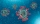The vaccination coverage of the population is 80%. Unvaccinated make up 60% of all infected. What percentage are unvaccinated more likely to be infected?
• Smoker male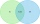For a person selected randomly from a certain population, events A and B are defined as follows. A = event the person is male B = event the person is a smoker. For this particular population, it is found that P(A ) = 0.53, P(B) = 0.15, and P(A n B ) = 0.1
• Poisson distribution - daisies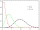The meadow behind FLD was divided into 100 equally large parts. Subsequently, it was found that there were no daisies in ten of these parts. Estimate the total number of daisies in the meadow. Assume that daisies are randomly distributed in the meadow.
• Three subjects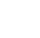In a class of 40 students, 18 passed mathematics, 19 passed accounts, 16 passed economics, 5 mathematics and accounts only, 6 mathematics only, 9 accounts only, 2 accounts and economics only, if each student offered at least one of the subjects. a) how ma
• A marriedA married couple planned to have 3 children. i. List the possible combinations of the sexes of 3 children. Use B for boy and G for girl. ii. Calculate the probability that all three children would be of the same gender
• A bagA bag contains 9 blue marbles and 1 green marble. What is the probability of drawing a blue marble followed by a green marble, without replacing the first marble before drawing the second marble.
• We rollWe roll two dice A. - what is the probability that the sum of the falling numbers is at most 4 B. - is at least 10 C. - is divisible by 5?
• SeedsFrom a box of spruce seeds with the germination of 80%, we randomly select 10 seeds and plant them. Find the median of the random variable: the number of germinating seeds.
• Two diceWe roll two dice. What is the probability that the sum of the falling numbers is greater than 3?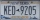A license plate has 3 letters followed by 4 numbers. Repeats are not allowed for the letters, but they are for the numbers. If they are issued at random, what is the probability that the 3 letters are in alphabetical order and the 3 numbers are consecutiv
• One dice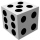Calculate the probability of one dice roll with the numbers 1, 2, 3, 4, 5, 6 on the walls. Write the results in a notebook in the shape of a fraction in the basic form: 2/3. a, The number 1 falls on the cube. b, The number 5 falls on the cube. c, An even
• Three diceThe player throwing the three dice asked G. Galilei the question: "Should I bet on the sum of 11 or the sum of 12?" What did Galilei answer him? Hint: write down all three triples of numbers that can be thrown and: have a total of 11 have a total of 12 an
• Playing cards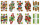From 32 playing cards containing 8 red cards, we choose 4 cards. What is the probability that just 2 will be red?
• Air draftThe numbers 1,2,3,4,5 are written on five tickets on the table. Air draft randomly shuffled the tickets and composed a 5-digit number from them. What is the probability that he passed: and, the largest possible number b, the smallest possible number c, a
• Six questions testThere are six questions in the test. There are 3 answers to each - only one is correct. In order for a student to take the exam, at least four questions must be answered correctly. Alan didn't learn at all, so he circled the answers only by guessing. What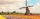How large must the group of people be so that the probability that two people have a birthday on the same day of the year is greater than 90%?
• Gold, silver, bronzeHow many ways can we divide gold, silver, bronze medals if 6 people are competing?
• The manufacturer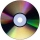The manufacturer found that 3% of the plates produced had a malfunction. Of the compliant, 75% are first and 25% second. What is the probability of producing first and second class plates?
• ProbabilityHow probable is a randomly selected three-digit number divisible by five or seven?

Do you have an exciting math question or word problem that you can't solve? Ask a question or post a math problem, and we can try to solve it.

We will send a solution to your e-mail address. Solved examples are also published here. Please enter the e-mail correctly and check whether you don't have a full mailbox.

Probability - math problems. Reason - math problems.